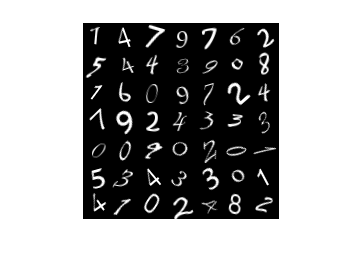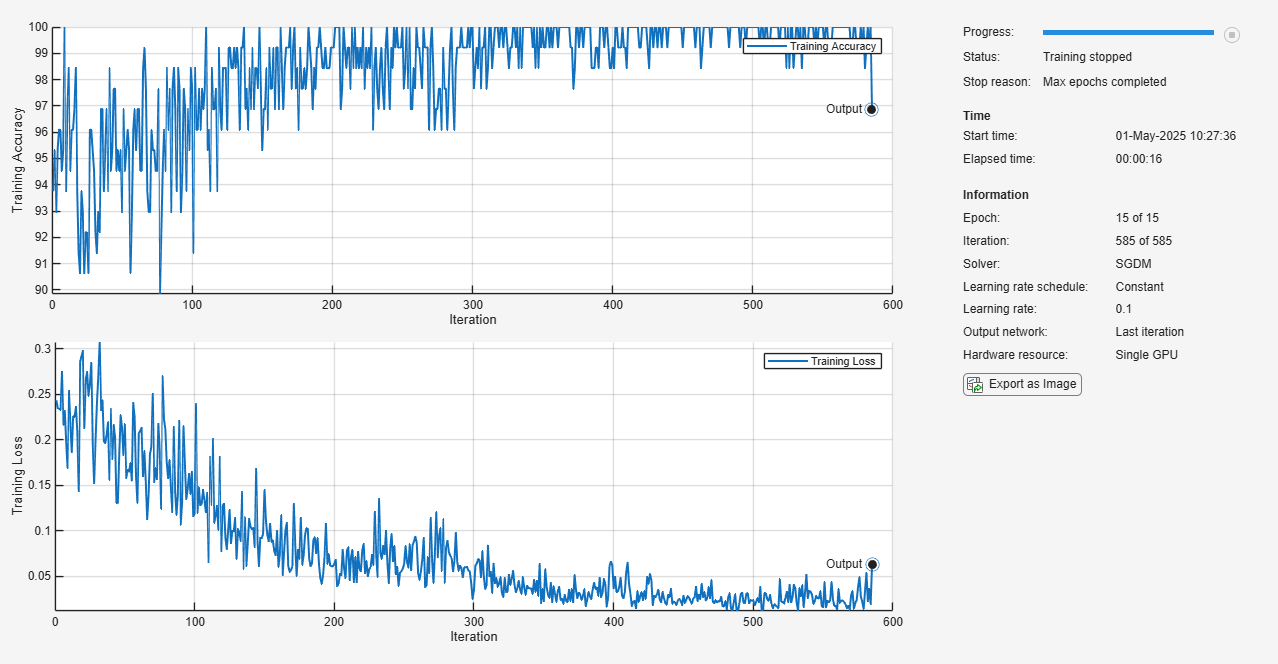# Resume Training from Checkpoint Network

This example shows how to save checkpoint networks while training a deep learning network and resume training from a previously saved network.

### Load Sample Data

Load the sample data as a 4-D array. `digitTrain4DArrayData` loads the digit training set as 4-D array data. `XTrain` is a 28-by-28-by-1-by-5000 array, where 28 is the height and 28 is the width of the images. 1 is the number of channels and 5000 is the number of synthetic images of handwritten digits. `YTrain` is a categorical vector containing the labels for each observation.

```[XTrain,YTrain] = digitTrain4DArrayData; size(XTrain)```
```ans = 1×4 28 28 1 5000 ```

Display some of the images in `XTrain`.

```figure; perm = randperm(size(XTrain,4),20); for i = 1:20 subplot(4,5,i); imshow(XTrain(:,:,:,perm(i))); end```### Define Network Architecture

Define the neural network architecture.

```layers = [ imageInputLayer([28 28 1]) convolution2dLayer(3,8,'Padding','same') batchNormalizationLayer reluLayer maxPooling2dLayer(2,'Stride',2) convolution2dLayer(3,16,'Padding','same') batchNormalizationLayer reluLayer maxPooling2dLayer(2,'Stride',2) convolution2dLayer(3,32,'Padding','same') batchNormalizationLayer reluLayer averagePooling2dLayer(7) fullyConnectedLayer(10) softmaxLayer classificationLayer];```

### Specify Training Options and Train Network

Specify training options for stochastic gradient descent with momentum (SGDM) and specify the path for saving the checkpoint networks.

```checkpointPath = pwd; options = trainingOptions('sgdm', ... 'InitialLearnRate',0.1, ... 'MaxEpochs',20, ... 'Verbose',false, ... 'Plots','training-progress', ... 'Shuffle','every-epoch', ... 'CheckpointPath',checkpointPath);```

Train the network. `trainNetwork` uses a GPU if there is one available. If there is no available GPU, then it uses CPU. `trainNetwork` saves one checkpoint network each epoch and automatically assigns unique names to the checkpoint files.

`net1 = trainNetwork(XTrain,YTrain,layers,options);`### Load Checkpoint Network and Resume Training

Suppose that training was interrupted and did not complete. Rather than restarting the training from the beginning, you can load the last checkpoint network and resume training from that point. `trainNetwork` saves the checkpoint files with file names on the form `net_checkpoint__195__2018_07_13__11_59_10.mat`, where 195 is the iteration number, `2018_07_13` is the date, and `11_59_10` is the time `trainNetwork` saved the network. The checkpoint network has the variable name `net`.

Load the checkpoint network into the workspace.

`load('net_checkpoint__195__2018_07_13__11_59_10.mat','net')`

Specify the training options and reduce the maximum number of epochs. You can also adjust other training options, such as the initial learning rate.

```options = trainingOptions('sgdm', ... 'InitialLearnRate',0.1, ... 'MaxEpochs',15, ... 'Verbose',false, ... 'Plots','training-progress', ... 'Shuffle','every-epoch', ... 'CheckpointPath',checkpointPath);```

Resume training using the layers of the checkpoint network you loaded with the new training options. If the checkpoint network is a DAG network, then use `layerGraph(net)` as the argument instead of `net.Layers`.

`net2 = trainNetwork(XTrain,YTrain,net.Layers,options);`